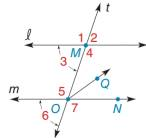Chapter 2.1, Problem 14E### Elementary Geometry for College St...

6th Edition
Daniel C. Alexander + 1 other
ISBN: 9781285195698

#### Solutions

Chapter
Section### Elementary Geometry for College St...

6th Edition
Daniel C. Alexander + 1 other
ISBN: 9781285195698
Textbook Problem
40 views

# Given: l ∥ m Transversal t m ∠ 1 = 4 x + 2 m ∠ 6 = 4 x − 2 Find: x and m ∠ 5Exercise 15, 16

To determine

To find:

The value of x and the measure of the given angle.

Explanation

The sum of angles that falls on the straight line is 180°.

Definition:

If there exists two parallel lines cut by a transversal then the pair of exterior angles on the same side of transversal is supplementary.

Given:

The given figure is,

lm cut by a transversal t.

The m1=4x+2 and m6=4x2.

Calculation:

Consider the given figure,

From the figure, lm with transversal t.

1 and 6 are exterior angles on the same side of transversal.

m1+m6=180°4x+2+4x2=180°8x=180°x=452

The value of x is 452.

Consider,

m1=4x+2…... (1)

Substitute 452 for x in equation (1)

### Still sussing out bartleby?

Check out a sample textbook solution.

See a sample solution

#### The Solution to Your Study Problems

Bartleby provides explanations to thousands of textbook problems written by our experts, many with advanced degrees!

Get Started

#### In Exercises 2340, find the indicated limit. 34. limx1x3+12x3+2

Applied Calculus for the Managerial, Life, and Social Sciences: A Brief Approach

#### In Exercises 5-8, graph the given function or equation. 2x3y=12

Finite Mathematics and Applied Calculus (MindTap Course List)

#### f(x) = (x2 3)2 has points of inflection at x = _____. a) 0,3,3 b) 1, 1 c) 0, 1, 1 d) 3,3

Study Guide for Stewart's Single Variable Calculus: Early Transcendentals, 8th

#### True or False: is monotonic.

Study Guide for Stewart's Multivariable Calculus, 8th